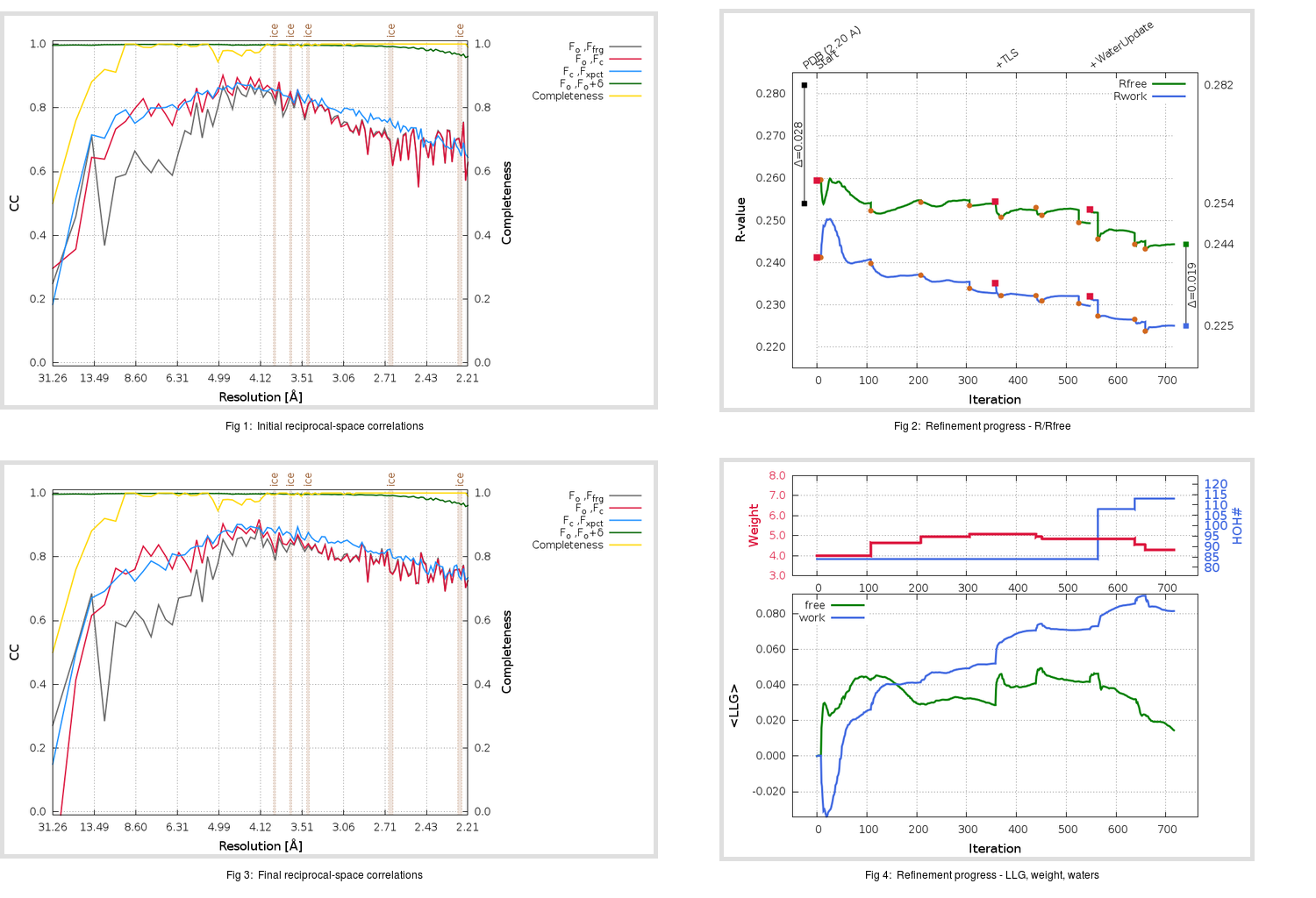Content:

```    Diffraction limits & principal axes of ellipsoid fitted to diffraction cut-off surface:
2.161         1.0000   0.0000   0.0000       0.894 a* - 0.447 b*
2.161         0.0000   1.0000   0.0000       b*
2.179         0.0000   0.0000   1.0000       c*
```

## Deposited

` `
 Date deposited Date data collection Resolution R, Rfree 20170214 20140621 2.20 0.2520 0.2820

Molprobity (CCP4 7.0 version) summary:

```Ramachandran outliers =   0.00 %
favored =  92.83 %
Rotamer outliers      =   2.49 %
C-beta deviations     =     0
Clashscore            =  10.48
RMS(bonds)            =   0.0043
RMS(angles)           =   1.04
MolProbity score      =   2.30
Resolution            =   2.20
R-work                =   0.2520
R-free                =   0.2820
```

```Number of waters      =    84

<B> (all atoms) =   54.86 ( sd =   15.82 ) for       2278 non-hydrogen atoms
<B>   (protein) =   54.57 ( sd =   15.90 ) for       2152 non-hydrogen atoms
<B>     (water) =   56.39 ( sd =   10.32 ) for         84 non-hydrogen atoms
<B>    (others) =   66.27 ( sd =   15.99 ) for         42 non-hydrogen atoms

B min/max       (all non-hydrogen atoms) =   28.70 /  135.73
B min/max   (protein non-hydrogen atoms) =   28.70 /  135.73
B min/max     (water non-hydrogen atoms) =   32.22 /   78.64
B min/max     (other non-hydrogen atoms) =   42.57 /   94.36
```

## BUSTER (re-)refinement

` `

Molprobity (CCP4 7.0 version) summary:

```Ramachandran outliers =   0.38 %
favored =  93.21 %
Rotamer outliers      =   4.98 %
C-beta deviations     =     0
Clashscore            =   4.89
RMS(bonds)            =   0.0111
RMS(angles)           =   1.66
MolProbity score      =   2.22
Resolution            =   2.20
R-work                =   0.2252
R-free                =   0.2445
```

```Number of waters      =   113

<B> (all atoms) =   47.36 ( sd =   10.53 ) for       2307 non-hydrogen atoms
<B>   (protein) =   46.82 ( sd =   10.29 ) for       2152 non-hydrogen atoms
<B>     (water) =   54.11 ( sd =   12.14 ) for        113 non-hydrogen atoms
<B>    (others) =   56.80 ( sd =    7.69 ) for         42 non-hydrogen atoms

B min/max       (all non-hydrogen atoms) =   12.33 /   88.87
B min/max   (protein non-hydrogen atoms) =   12.33 /   88.87
B min/max     (water non-hydrogen atoms) =   31.15 /   84.61
B min/max     (other non-hydrogen atoms) =   47.41 /   68.21
```

Refinement progression:Results:

` `
 File Remark 5X4S_aB_refine.01_03_refine.pdb.gz exact refinement commands are in header 5X4S_aB_refine.01_03_refine.mtz.gz including original deposited data and several re-refinement map coefficients 5X4S_aB_refine.01_03_BUSTER_model.cif.gz including any non-standard compound restraints 5X4S_aB_refine.01_03_BUSTER_refln.cif.gz